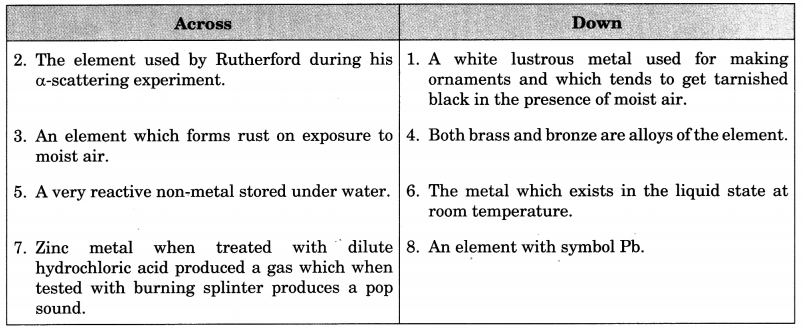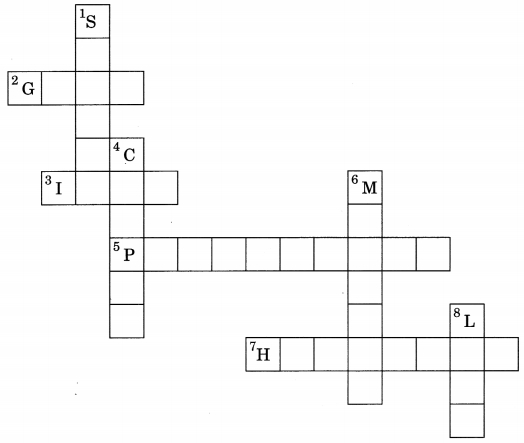# MCQ Questions for Class 9 Science Chapter 3 Atoms and Molecules with Answers

Check the below NCERT MCQ Questions for Class 9 Science Chapter 3 Atoms and Molecules with Answers Pdf free download. MCQ Questions for Class 9 Science with Answers were prepared based on the latest exam pattern. We have Provided Atoms and Molecules Class 9 Science MCQs Questions with Answers to help students understand the concept very well. https://mcqquestions.guru/mcq-questions-for-class-9-science-chapter-3/

You can refer to NCERT Solutions for Class 9 Science Chapter 3 Atoms and Molecules to revise the concepts in the syllabus effectively and improve your chances of securing high marks in your board exams.

## Atoms and Molecules Class 9 MCQs Questions with Answers

Atoms And Molecules Class 9 MCQ Question 1.
The chemical symbol for nitrogen gas is
(a) Ni
(b) N2
(c) N+
(d) N

Atoms And Molecules Class 9 MCQ With Answers Question 2.
The chemical symbol for sodium is
(a) So
(b) Sd
(c) NA
(d) Na

Class 9 Science Chapter 3 MCQ Question 3.
Which of the following would weigh the highest?
(a) 0.2 mole of sucrose (C12H22O11)
(b) 2 moles of CO2
(c) 2 moles of CaCO3
(d) 10 moles of H2O

Answer: (c) 2 moles of CaCO3

Class 9 Atoms And Molecules MCQ Question 4.
Which of the following has maximum number of atoms?
(a) 18 g of H2O
(b) 18 g of O2
(c) 18 g of CO2
(d) 18 g of CO4

Answer: (d) 18 g of CO4

Atom And Molecules Class 9 MCQ Question 5.
Which of the following correctly represents 360 g of water?
(i) 2 moles of H2O
(ii) 20 moles of water
(iii) 6.022 × 1023 molecules of water
(iv) 1.2044 × 1025 molecules of water
(a) (i)
(b) (i) and (iv)
(c) (ii) and (iii)
(d) (ii) and (iv)

MCQ On Atoms And Molecules Class 9 Question 6.
3.42 g of sucrose are dissolved in 18 g of water in a beaker. The number of oxygen atoms in the solution are
(a) 6.68 × 1023
(b) 6.09 × 1022
(c) 6.022 × 1023
(d) 6.022 × 102321

MCQ Of Atoms And Molecules Of Class 9 Question 7.
A change in the physical state can be brought about
(a) only when energy is given to the system
(b) only when energy is taken out from the system
(c) when energy is either given to, or taken out from the system
(d) without any energy change

Answer: (c) when energy is either given to, or taken out from the system

Class 9 Science Ch 3 MCQ Question 8.
The relative molecular mass of Na2S2O3.5H2O is
(a) 250 amu
(b) 250 g
(c) 248 amu
(d) 248 g

MCQ Atoms And Molecules Class 9 Question 9
Which of the following has maximum number of atom?
(a) 18 g H2O
(b) 18 g of O2
(c) 18 g of CO2
(d) 18 g of CH4

Answer: (d) 18 g of CH4

Ch 3 Science Class 9 MCQ Question 10.
Percentage of calcium in calcium carbonate is
(a) 40
(b) 30
(c) 48
(d) 36

Atoms And Molecules MCQ Class 9 Question 11.
Which has maximum number of molecules?
(a) 1 g of CO2
(b) 1 g of N2
(c) 1 g of H2
(d 1 g of CH4

Answer: (c) 1 g of H2

Class 9 Chemistry Chapter 3 MCQ Question 12.
What mass of carbon dioxide (CO2) will contain 3.011 × 1023 molecules?
(a) 11.0 g
(b) 22.0 g
(c) 4.4 g
(d) 44.0 g

Class 9 Chapter 3 Science MCQ Question 13.
The value of Avogadro’s constant is:
(a) 6.0 × 1024
(b) 6.01 × 1022
(c) 6.022 × 1023
(d) 6.022 × 10-23

Class 9 Science Chapter 3 MCQ With Answers Question 14.
How many times an atom of sulphur is heavier than an atom of carbon?
(a) 32 times
(b) 12 times
(c) 8/3 times
(d) 12/32 times

Chapter 3 Science Class 9 MCQ Question 15.
The number of oxygen atoms in 4.4 g of CO2 is approx.
(a) 6 × 1022
(b) 6
(c) 12 × 1023
(d) 1.2 × 1023

Question 16.
Which of the following represents 12 u?
(a) Mass of 1 hydrogen atom
(b) Mass of C-12 atom
(c) Mass of 0-16 atom
(d) 1/12th of mass of C-12 atom.

Answer: (d) 1/12th of mass of C-12 atom.

Question 17.
Which of the following would weigh the highest?
(a) 0.2 mole of sucrose (C12H22O11)
(b) 2 moles of CO2
(c) 2 moles of CaCO3
(d) 10 moles of H2O

Answer: (c) 2 moles of CaCO3

Question 18.
The molecule having an atomicity of 4 is:
(a) Sulphate molecule
(b) Ozone molecule
(c) Phosphorus molecule
(d) Methane molecule

Fill in the blanks

1. In a chemical reaction, the sum of the masses of the reactants and products remain unchanged. This is called ……………

Answer: Law of Conservation of Mass

2. A group of atoms carrying a fixed charge on them is called …………..

3. The formula unit mass of Ca3(PO4)2 is ……………

Answer: (3 × atomic mass of calcium) + (2 × atomic mass of phosphorus) + (8 × atomic mass of oxygen) = 310

4. Formula of sodium carbonate is ………….. and that of ammonium sulphate is ……………

5. Elements are represented by symbols and molecules by …………….

6. The combining power of an element is known as its …………….

7. An atom or a group of atoms which carries positive or negative charge is called an ……………..

8. The volume occupied by one mole of a gas under standard conditions of temperature and pressure is called ……………..

9. The number of atoms present in one molecule of the substance is called its ……………

10. Atomicity of H2SO4 is …………….

Match the following columns
1.

 Column I Column II (a) 28 g of He (i) 58.54 (b) 0.5 mole of O2 (ii) 7 mol (c) Molecular mass of common salt (iii) 60 g (d) 1.5 mole of Ca (iv) 16 g

 Column I Column II (a) 28 g of He (ii) 7 mol (b) 0.5 mole of O2 (iv) 16 g (c) Molecular mass of common salt (i) 58.54 (d) 1.5 mole of Ca (iii) 60 g

2.

 Column I Column II (a) Sodium carbonate (i) NaHCO3 (b) Sodium bicarbonate (ii) Na3PO4 (c) Sodium sulphate (iii) Na2CO3 (d) Sodium phosphate (iv) Na2SO4

 Column I Column II (a) Sodium carbonate (iii) Na2CO3 (b) Sodium bicarbonate (i) NaHCO3 (c) Sodium sulphate (iv) Na2SO4 (d) Sodium phosphate (ii) Na3PO4

3.

 Column I Column II (a) Silver (i) Na (b) Sodium (ii) Ag (c) Gold (iii) K (d) Potassium (iv) Au

 Column I Column II (a) Silver (ii) Ag (b) Sodium (i) Na (c) Gold (iv) Au (d) Potassium (iii) K

Answer the questions in one word:

1. Smallest particle which cannot be divided into simpler parts.

2. Which experiment led to the discovery of the atomic nucleus?

Answer: Rutherford’s alpha particle scattering experiment

3. What is the mass of a proton and a neutron?

4. What do the species $$_{1}^{3}$$A and $$_{2}^{3}$$B represent?

5. What is the formula of hydrogen carbonate?

6. What is the chemical formula of aluminium sulphate?

7. What is the formula unit mass of CaCl2?

8. What is the mass of 5 moles H2O?

1. Complete the following crossword puzzle by using the name of the chemical elements. Use the data given in table mentioned below.Down:
2. Gold
3. Iron
5. Phosphorus
7. Hydrogen

Across:
1. Silver
4. Copper
6. Mercury